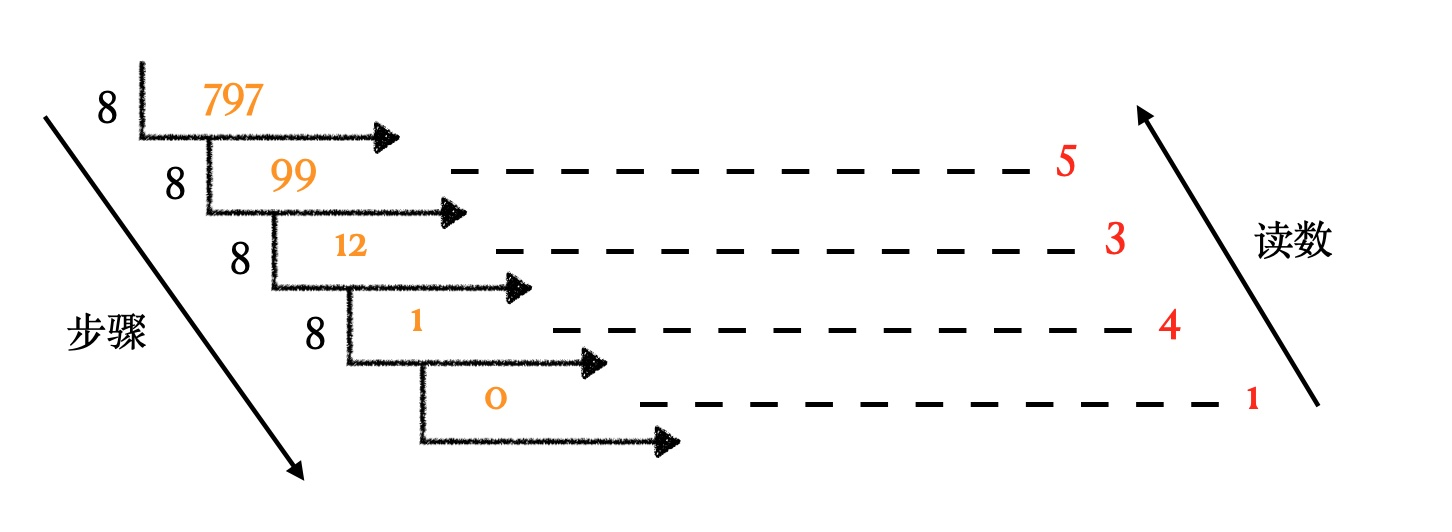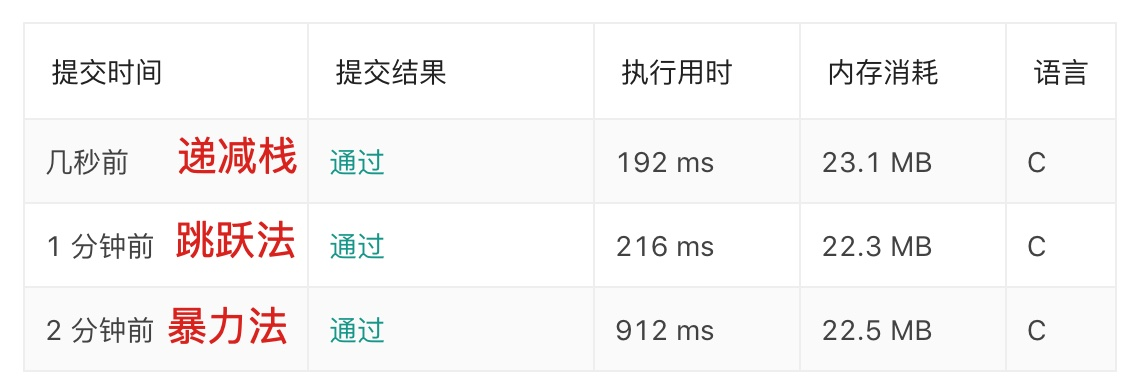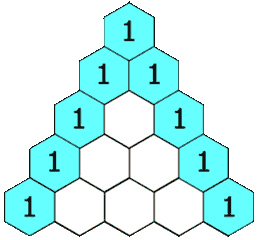# 数据结构与算法 - 栈应用## 进制转换``void conversion(int N){    SqStack S;    SElemType e;    //1.初始化一个空栈S    InitStack(&S);    //2.每次取余数直到结果为0为止，把每次的余数进栈    while (N) {        PushData(&S, N%8);        N = N/8;    }    //3.出栈得到我们想要的十进制数据    while (!StackEmpty(S)) {        Pop(&S, &e);        printf("%d\n",e);    }}``

## 每日温度

### 暴力法

``int  *dailyTemperatures_1(int* T, int TSize, int* returnSize){    int *result = (int *)malloc(sizeof(int) * TSize);    *returnSize = TSize;    result[TSize-1] = 0;    for(int i = 0;i < TSize-1;i++)        if(i>0 && T[i] == T[i-1])            result[i] = result[i-1] == 0?0:result[i-1]-1;        else{            for (int j = i+1; j < TSize; j++) {                if(T[j] > T[i]){                    result[i] = j-i;                    break;                }                if (j == TSize-1) {                    result[i] = 0;                }            }        }    return result;}``

### 倒序跳跃法

1. 从右到左遍历. 因为最后一天的气温不会再升高,默认等于0
2. i 从[TSize-2,0]; 从倒数第二天开始遍历比较. 每次减一
3. j 从[i+1,TSize]遍历, j+=result[j],可以利用已经有结果的位置进行跳跃,从而减少遍历次数
1. 若T[i]<T[j],那么result = j - i
2. 若reuslt[j] == 0,则表示后面不会有更大的值,那么当前值就应该也是0
``int  *dailyTemperatures_2(int* T, int TSize, int* returnSize){    int *result = (int *)malloc(sizeof(int) * TSize);    *returnSize = TSize;    result[TSize-1] = 0;    for (int i=TSize-2; i >= 0; i--) {        for (int j = i+1; j < TSize; j+=result[j]) {            if (T[i] < T[j]) {                result[i] = j-i;                break;            }else            {                if (result[j] == 0) {                    result[i] = 0;                    break;                }            }        }    }    return result;}``

### 单调递减栈

1. 初始化一个栈,栈中存储的是元素的索引值index

2. 遍历整个温度数组从[0,TSize]

1. 如果当前的栈为空,则直接入栈
2. 如果当前的元素小于栈顶元素,则入栈
3. 如果栈顶元素<当前元素,则将当前元素索引index-栈顶元素index,计算完毕则将当前栈顶元素移除,将当前元素索引index 存储到栈中; 出栈后,只要栈不为空.继续比较,直到栈顶元素不能满足T[i] > T[stack_index[top-1]]
4. while循环结束后,当前元素也需要入栈
``int* dailyTemperatures_3(int* T, int TSize, int* returnSize) {    int* result = (int*)malloc(sizeof(int)*TSize);    // 用栈记录T的下标。    int* stack_index = malloc(sizeof(int)*TSize);    *returnSize = TSize;    // 栈顶指针。    int top = 0;    int tIndex;    for (int i = 0; i < TSize; i++)        result[i] = 0;    for (int i = 0; i < TSize; i++) {        // 若当前元素大于栈顶元素，栈顶元素出栈。即温度升高了，所求天数为两者下标的差值。        while (top > 0 && T[i] > T[stack_index[top-1]]) {            tIndex = stack_index[top-1];            result[tIndex] = i - tIndex;            top--;        }        // 当前元素入栈。        stack_index[top] = i;        top++;    }    return result;}``## 括号匹配

`` 1// 括号匹配 2Status IsCorrect(char *string) { 3    Stack S; 4    InitStack(&S); 5 6    int i = 0; 7    char temp = string; 8    while (temp) { 9        printf("string is %c \n",temp);10        if (temp == '[') {11            PushData(&S, '[');12        } else if (temp == '(') {13            PushData(&S, '(');14        } else if (temp == ')') {15            char top;16            GetTop(S, &top);17            if (top == '(') {18                Pop(&S, &top);19            } else {20                PushData(&S, ')');21                // return FALSE;22            }23        } else if (temp == ']') {24            char top;25            GetTop(S, &top);26            if (top == '[') {27                Pop(&S, &top);28            } else {29                PushData(&S, ']');30                // return FALSE;31            }32        }33        i ++;34        temp = string[i];35    }3637    return IsEmpty(S)?SUCCESS:FALSE;38}``

## 杨辉三角``int** generate(int numRows, int* returnSize){    *returnSize = numRows;    int **res = (int **)malloc(sizeof(int*)*numRows);    for (int i = 0; i < numRows; i++) {        res[i] = (int *)malloc(sizeof(int)*(i+1));        res[i] = 1;        res[i][i] = 1;        for (int j = 1; j < i; j++) {            res[i][j] = res[i-1][j] + res[i-1][j-1];        }    }    return res;}``

## 爬楼梯

### 递归

• `n-1` 阶爬 1 个台阶
• `n-2` 阶爬 2个台阶

``int ClimbStairs(int n){    if (n<1)  return 0;    if (n == 1) return 1;    if (n == 2) return 2;    return ClimbStairs(n-1) + ClimbStairs(n-2);}``

### 动态规划

``int ClimbStairs(int n){    if(n==1) return 1;    int temp = n+1;    int *sum = (int *)malloc(sizeof(int) * (temp));    sum = 0;    sum = 1;    sum = 2;    for (int i = 3; i <= n; i++) {        sum[i] = sum[i-1] + sum[i-2];    }    return sum[n];}``

## 字符串编码

s = “3[a]2[bc]”,返回 “aaabcbc”
s = “3[a2[c]]” 返回 “accaccacc”
s = “2[abc]3[cd]ef” 返回 “abcabccdcdcdef”

1. 遍历字符串 S,如果当前字符不为方括号”]” 则入栈stack中
2. 如果当前字符遇到了方括号”]” 则:
1. 首先找到要复制的字符,例如stack=”12[a”,那么我要首先获取字符a;将这个a保存在另外一个栈去tempStack
2. 接下来,要找到需要备份的数量,例如stack=”12[a”,因为出栈过字符”a”,则当前的top指向了”[“,也就是等于2
3. 而12对于字符串是2个字符, 我们要通过遍历找到数字12的top上限/下限的位置索引, 此时上限curTop = 2, 下限通过出栈,top = -1
4. 根据范围[-1,2],读取出12保存到strOfInt 字符串中来, 并且将字符”12\0”,转化成数字12
5. 当前top=-1,将tempStack中的字符a,复制12份入栈到stack中来
6. 为当前的stack扩容, 在stack字符的末尾添加字符结束符合’\0’
``char * decodeString(char * s){    /*.     1.获取字符串长度     2.设置默认栈长度50     3.开辟字符串栈(空间为50)     4.设置栈头指针top = -1;     */    int len = (int)strlen(s);    int stackSize = 50;    char* stack = (char*)malloc(stackSize * sizeof(char));    int top = -1;    //遍历字符串,在没有遇到"]" 之前全部入栈    for (int i = 0; i < len; ++i) {        if (s[i] != ']') {            //优化:如果top到达了栈的上限,则为栈扩容;            if (top == stackSize - 1) {                stack = realloc(stack, (stackSize += 50) * sizeof(char));            }            //将字符入栈stack            stack[++top] = s[i];            printf("#① 没有遇到']'之前# top = %d\n",top);        }        else {            int tempSize = 10;            char* temp = (char*)malloc(tempSize * sizeof(char));            int topOfTemp = -1;            printf("#② 开始获取要复制的字符信息之前 # top = %d\n",top);            //从栈顶位置开始遍历stack,直到"["结束;            //把[a]这个字母a 赋值到temp栈中来;            //简单说,就是将stack中方括号里的字符出栈,复制到temp栈中来;            while (stack[top] != '[') {                //优化:如果topOfTemp到达了栈的上限,则为栈扩容;                if (topOfTemp == tempSize - 1) {                    temp = realloc(temp, (tempSize += 10) * sizeof(char));                }                //temp栈的栈顶指针自增;                ++topOfTemp;                //将stack栈顶字符复制到temp栈中来;                temp[topOfTemp] = stack[top];                //stack出栈,则top栈顶指针递减;                top--;            }            printf("#② 开始获取要复制的字符信息之后 # top = %d\n",top);            //找到倍数数字.strOfInt字符串;            //注意:如果是大于1位的情况就处理            char strOfInt;            //p记录当前的top;            int curTop = top;            printf("#③ 开始获取数字,数字位置上限 # curTop = %d\n",curTop);            //top--的目的是把"["剔除,才能找到数字;            top--;            //遍历stack得出数字            //例如39[a] 就要找到这个数字39.            //p指向当前的top,我就知道上限了; 那么接下来通过循环来找它的数字下限;            //结束条件:栈指针指向为空! stack[top] 不等于数字            while (top != -1 && stack[top] >= '0' && stack[top] <= '9') {                top--;            }            printf("#③ 开始获取数字,数字位置下限 # top = %d\n",top);            //从top-1遍历到p之间, 把stack[top-1,p]之间的数字复制到strOfInt中来;            //39中3和9都是字符. 我们要获取到这2个数字,存储到strOfInt数组            for (int j = top + 1; j < curTop; ++j) {                strOfInt[j - (top + 1)] = stack[j];            }            //为字符串strOfInt数组加一个字符结束后缀'\0'            strOfInt[curTop - (top + 1)] = '\0';            //把strOfInt字符串转换成整数 atoi函数;            //把字母复制strOfInt份到stack中去;            //例如39[a],就需要把复制39份a进去;            int curNum = atoi(strOfInt);            for (int k = 0; k < curNum ; ++k) {                //从-1到topOfTemp 范围内,复制curNum份到stackTop中去;                int kk = topOfTemp;                while (kk != -1) {                    //优化:如果stack到达了栈的上限,则为栈扩容;                    if (top == stackSize - 1) {                        stack = realloc(stack, (stackSize += 50) * sizeof(char));                    }                    //将temp栈的字符复制到stack中;                    //stack[++top] = temp[kk--];                    ++top;                    stack[top] = temp[kk];                    kk--;                }            }            free(temp);            temp = NULL;        }    }    //realloc 动态内存调整;    //void *realloc(void *mem_address, unsigned int newsize);    //构成字符串stack后, 在stack的空间扩容.    char* ans = realloc(stack, (top + 1) * sizeof(char));    ans[++top] = '\0';    //stack 栈不用,则释放;    free(stack);    return ans;}``

## 去除重复字母

1. 创建一个数组保存每个字母出现的次数，很显然只需要创建一个 `26` 个容量的数组即可
2. 遍历字符串，如果栈是空直接入栈，否则进行判断：
1. 如果栈中存在相同的字符，直接对相应字符存在的数量进行减一处理
2. 如果当前字符大于栈顶元素，那么直接入栈
3. 如果当前字符小于栈顶元素，继续判断栈顶元素后面是否还有，如果有那么出栈直到栈顶当前字符大于栈顶元素。
``char *MinString(char *string) {    // 边界判断    if (string == NULL || strlen(string) == 0) return "";    if (strlen(string) == 1) return string;    // 用一个数组保存每个字母出现的次数    int record = {0};    unsigned long len = strlen(string);    int top = -1;    char *stack = (char *)malloc(len*sizeof(char)+1);    stack = memset(stack, 0, len*sizeof(char)+1);    for (int i = 0; i < len; i++) {        record[string[i]-'a']++;    }    for (int i = 0; i < len; i++) {        // 判断当前字符是否已经在栈中        int exist = 0;        for (int j = 0; j <= top; j ++) {            if (stack[j] == string[i]) {                exist = 1;            }        }        if (exist == 1) {// 如果存在直接对数组中相应字母数量减一            record[string[i]-'a']--;        } else {            // 如果栈为空或者栈顶小于当前元素直接入栈，并减少数量            if (top == -1 || stack[top] < string[i]) {                top++;                stack[top] = string[i];                record[string[i]-'a']--;            } else {                // 否则一直出栈直到栈顶元素小于当前元素                while (top>=0 && record[stack[top]-'a'] > 0 && stack[top] > string[i]) {                    top--;                }                // 入栈新元素，并减少数量                stack[++top] = string[i];                record[string[i]-'a']--;            }        }    }    // 字符串末尾补\0    stack[++top] = '\0';    return stack;}``

## 总结

------------- 本文结束  感谢您的阅读 -------------